## Vlookup and return multiple values based on one or multiple criteria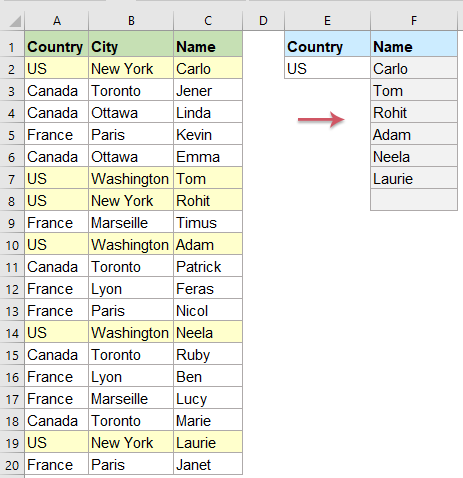Normally, you can use the Vlookup function to get the first corresponding value, but, sometimes, you want to return all matching records based on a specific criterion. This article, I will talk about how to vlookup and return all matching values vertically, horizontally or into one single cell.

Vlookup and return all corresponding values vertically

Vlookup and return all corresponding values horizontally

Vlookup and return all corresponding values into one cell

#### Vlookup and return all corresponding values vertically

To return all matching values vertically based on a specific criterion, please apply the following array formula:

1. Enter or copy this formula into a blank cell where you want to output the result:

=IFERROR(INDEX(\$C\$2:\$C\$20, SMALL(IF(\$E\$2=\$A\$2:\$A\$20, ROW(\$A\$2:\$A\$20)-ROW(\$A\$2)+1), ROW(1:1))),"" )

Note: In the above formula, C2:C20 is the column contains the matching record you want to return; A2:A20 is the column contains the criterion; and E2 is the specific criterion that you want to return values based on. Please change them to your need.

2. Then, press Ctrl + Shift + Enter keys together to get the first value, and then drag the fill handle down to get all the corresponding records as you need, see screenshot: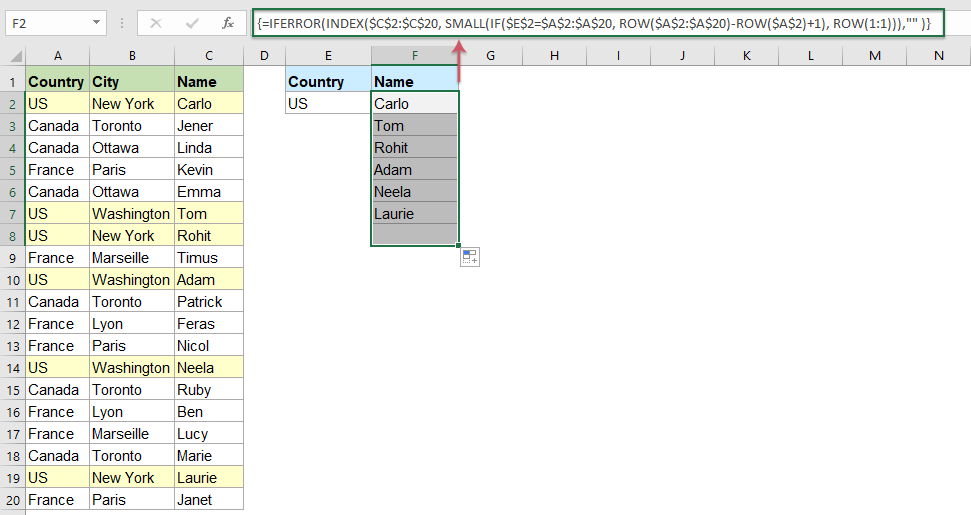Tips:

To Vlookup and return all matching values based on more specific values vertically, please apply the below formula, and press Ctrl + Shift + Enter keys.

=IFERROR(INDEX(\$C\$2:\$C\$20, SMALL(IF(1=((--(\$E\$2=\$A\$2:\$A\$20))*(--(\$F\$2=\$B\$2:\$B\$20))), ROW(\$A\$2:\$A\$20)-ROW(\$A\$2)+1), ROW(1:1))),"" )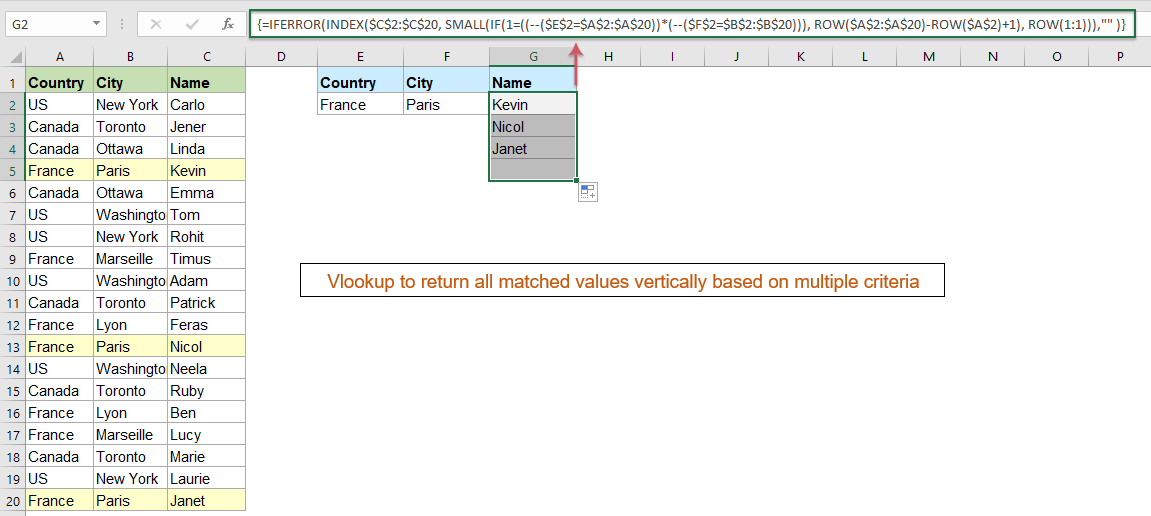#### Vlookup and return all corresponding values horizontally

If you want to get the matching values displayed in horizontal order, the below array formula can help you.

1. Enter or copy this formula into a blank cell where you want to output the result:

=IFERROR(INDEX(\$C\$2:\$C\$20,SMALL(IF(\$F\$1=\$A\$2:\$A\$20,ROW(\$A\$2:\$A\$20)-ROW(\$A\$2)+1),COLUMN(A1))),"")

Note: In the above formula, C2:C20 is the column contains the matching record you want to return; A2:A20 is the column contains the criterion; and F1 is the specific criterion that you want to return values based on. Please change them to your need.

2. Then, press Ctrl + Shift + Enter keys together to get the first value, and then drag the fill handle right to get all the corresponding records as you need, see screenshot: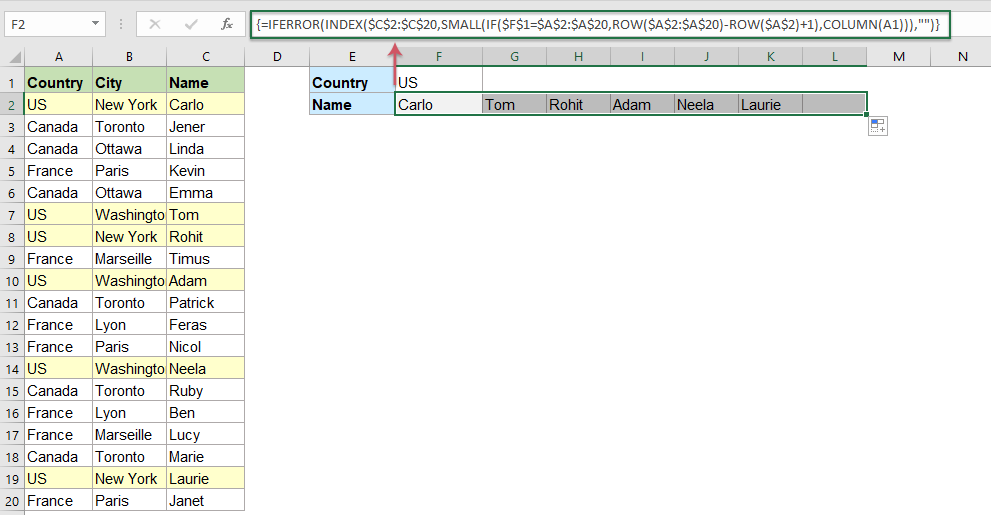Tips:

To Vlookup and return all matching values based on more specific values horizontally, please apply the below formula, and press Ctrl + Shift + Enter keys.

=IFERROR(INDEX(\$C\$2:\$C\$20,SMALL(IF(1=((--(\$F\$1=\$A\$2:\$A\$20))*(--(\$F\$2=\$B\$2:\$B\$20))),ROW(\$A\$2:\$A\$20)-ROW(\$A\$2)+1),COLUMN(A1))),"")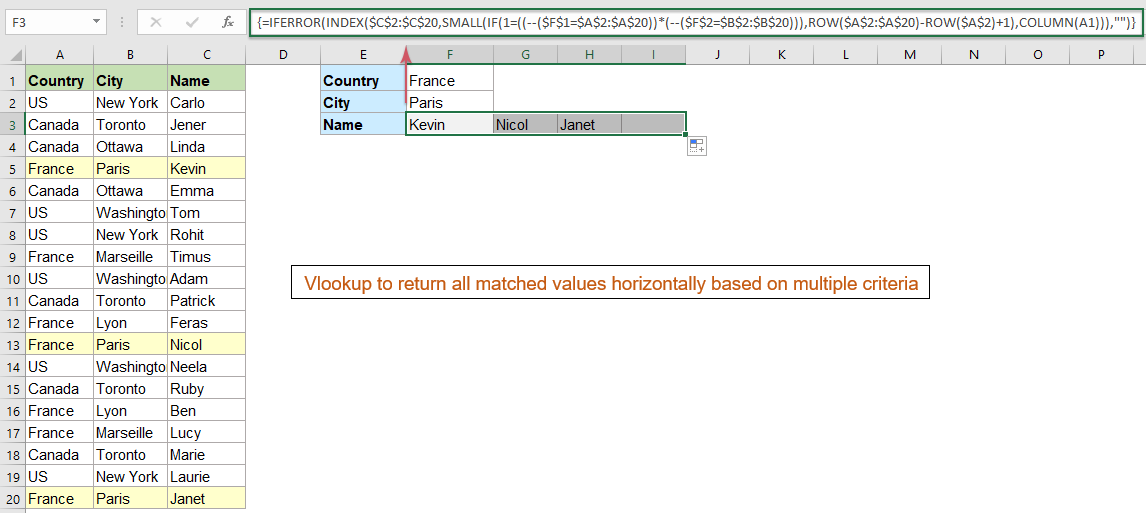#### Vlookup and return all corresponding values into one cell

To vlookup and return all corresponding values into a single cell, you should apply the following array formula.

1. Enter or copy below formula into a blank cell:

=TEXTJOIN(", ",TRUE,IF(\$A\$2:\$A\$20=F1,\$C\$2:\$C\$20,""))

Note: In the above formula, C2:C20 is the column contains the matching record you want to return; A2:A20 is the column contains the criterion; and F1 is the specific criterion that you want to return values based on. Please change them to your need.

2. Then, press Ctrl + Shift + Enter keys together to get all matching values into a single cell, see screenshot: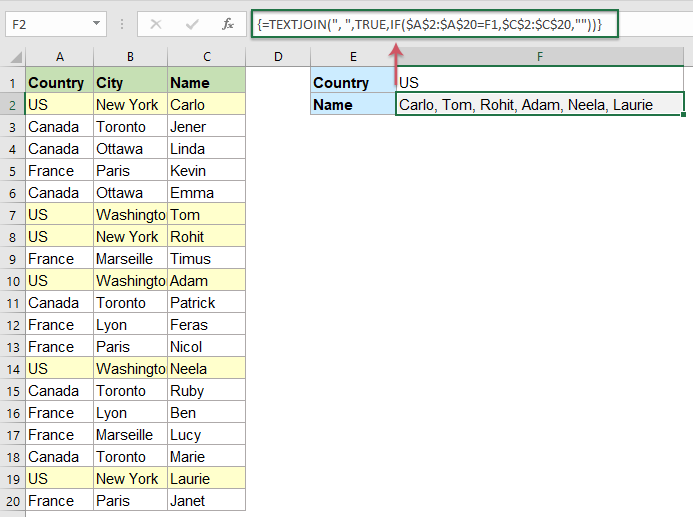Tips:

To Vlookup and return all matching values based on more specific values in a single cell, please apply the below formula, and press Ctrl + Shift + Enter keys.

=TEXTJOIN(", ",TRUE,IF((\$A\$2:\$A\$20=F1)*(\$B\$2:\$B\$20=F2),\$C\$2:\$C\$20,""))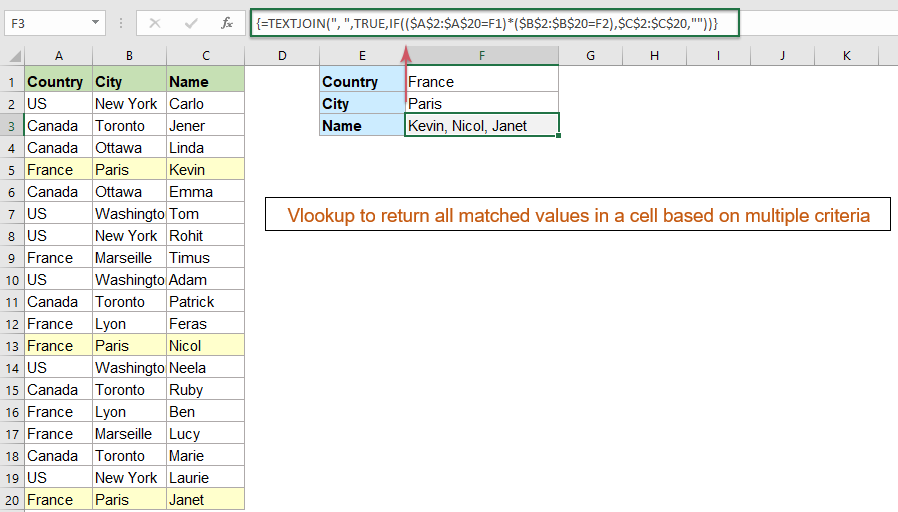Note: This formula only applied successfully in Excel 2016 and later versions. If you don’t have Excel 2016, please view here to get it down.

#### More relative Vlookup articles:

• Vlookup And Return Multiple Values From Drop Down List
• In Excel, how could you vlookup and return multiple corresponding values from a drop down list, which means when you choose one item from the drop down list, all of its relative values are displayed at once as following screenshot shown. This article, I will introduce the solution step by step.
• Vlookup To Return Blank Instead Of 0 Or N/A In Excel
• Normally, when you apply the vlookup function to return the corresponding value, if your matching cell is blank, it will return 0, and if your matching value is not found, you will get an error #N/A value. Instead of displaying the 0 or #N/A value, how can you make it show blank cell?
• Vlookup To Return Multiple Columns From Excel Table
• In Excel worksheet, you can apply the Vlookup function to return the matching value from one column. But, sometimes, you may need to extract matched values from multiple columns as following screenshot shown. How could you get the corresponding values at the same time from multiple columns by using the Vlookup function?
• Vlookup Values Across Multiple Worksheets
• In excel, we can easily apply the vlookup function to return the matching values in a single table of a worksheet. But, have you ever considered that how to vlookup value across multiple worksheet? Supposing I have the following three worksheets with range of data, and now, I want to get part of the corresponding values based on the criteria from these three worksheets.

## The Best Office Productivity Tools

### Kutools for Excel Solves Most of Your Problems, and Increases Your Productivity by 80%

• Super Formula Bar (easily edit multiple lines of text and formula); Reading Layout (easily read and edit large numbers of cells); Paste to Filtered Range...
• Merge Cells/Rows/Columns and Keeping Data; Split Cells Content; Combine Duplicate Rows and Sum/Average... Prevent Duplicate Cells; Compare Ranges...
• Select Duplicate or Unique Rows; Select Blank Rows (all cells are empty); Super Find and Fuzzy Find in Many Workbooks; Random Select...
• Exact Copy Multiple Cells without changing formula reference; Auto Create References to Multiple Sheets; Insert Bullets, Check Boxes and more...
• Favorite and Quickly Insert Formulas, Ranges, Charts and Pictures; Encrypt Cells with password; Create Mailing List and send emails...
• Extract Text, Add Text, Remove by Position, Remove Space; Create and Print Paging Subtotals; Convert Between Cells Content and Comments...
• Super Filter (save and apply filter schemes to other sheets); Advanced Sort by month/week/day, frequency and more; Special Filter by bold, italic...
• Combine Workbooks and WorkSheets; Merge Tables based on key columns; Split Data into Multiple Sheets; Batch Convert xls, xlsx and PDF...
• Pivot Table Grouping by week number, day of week and more... Show Unlocked, Locked Cells by different colors; Highlight Cells That Have Formula/Name...### Office Tab - brings tabbed interface to Office, and make your work much easier

• Enable tabbed editing and reading in Word, Excel, PowerPoint, Publisher, Access, Visio and Project.
• Open and create multiple documents in new tabs of the same window, rather than in new windows.
• Increases your productivity by 50%, and reduces hundreds of mouse clicks for you every day!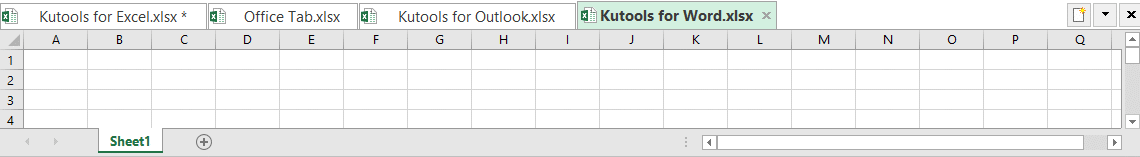No ratings yet. Be the first to rate!
This comment was minimized by the moderator on the site
gents

would you please share with me the formula of the three ways but for data in horizontal table.
This comment was minimized by the moderator on the site
Hello, Ahmed,
Get the results vertically: =IFERROR(INDEX(\$B\$2:\$K\$2, SMALL(IF(\$A\$5=\$B\$1:\$K\$1, COLUMN(\$B\$1:\$K\$1:\$K\$1)-COLUMN(\$B\$1)+1), ROW(1:1))),"" )
Get the results horizontally: =IFERROR(INDEX(\$B\$2:\$K\$2, SMALL(IF(\$D\$5=\$B\$1:\$K\$1, COLUMN(\$B\$1:\$K\$1:\$K\$1)-COLUMN(\$B\$1)+1), COLUMN(A1))),"" )
Ge the results in one cell: =TEXTJOIN(", ",TRUE,IF(\$B\$1:\$K\$1=J5,\$B\$2:\$K\$2,""))
Note: These formulas are array formulas, you should press Ctrl + Shift + Enter keys together to get the correct result.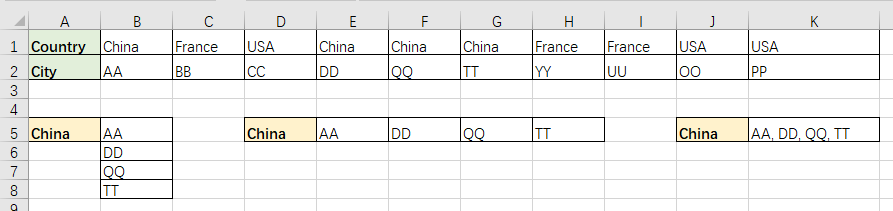This comment was minimized by the moderator on the site
thanks for your greet support and quick response
This comment was minimized by the moderator on the site
how do u get all rows to fill up like in yr video without pressing ctrl+shift+enter
This comment was minimized by the moderator on the site
After I extend the data, some of the cells is showing a "0" instead of blank
This comment was minimized by the moderator on the site
It means that there is missing data or the wrong type of data in the cell(s) from the column that is being referrenced. For instance, Im working with a bunch of data and I want all ID numbers for workers under a certain supervisor. However, in the table column being referenced with all workers' ID numbers, some cells had the workers last name in it and not their ID numbers. Those exact cells produced 0s when the entire formula was put in, and this is because it was text within a column that is mostly numbers.
This comment was minimized by the moderator on the site
How can you tweak the formula so that you have multiple outputs in column D? E.g. I want to populate column D with each of the countries, such that all of their respective cities will be returned in E? The only work around I have can see is manually changing \$D\$2
This comment was minimized by the moderator on the site
Thank you very much, you save lots of work here!

I changed a little : (=TEXTJOIN(CHAR(10),TRUE,IF(\$A\$2:\$A\$15=D2,\$B\$2:\$B\$15,""))}

Regards,

Jeff
There are no comments posted here yet